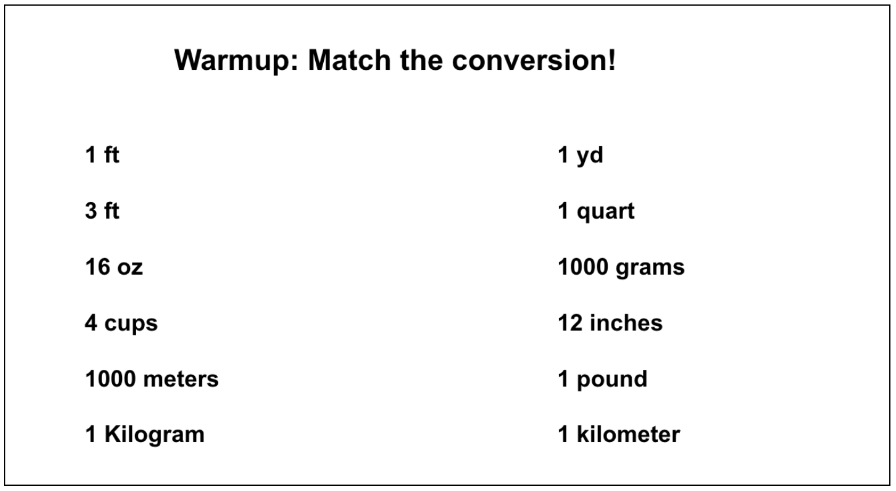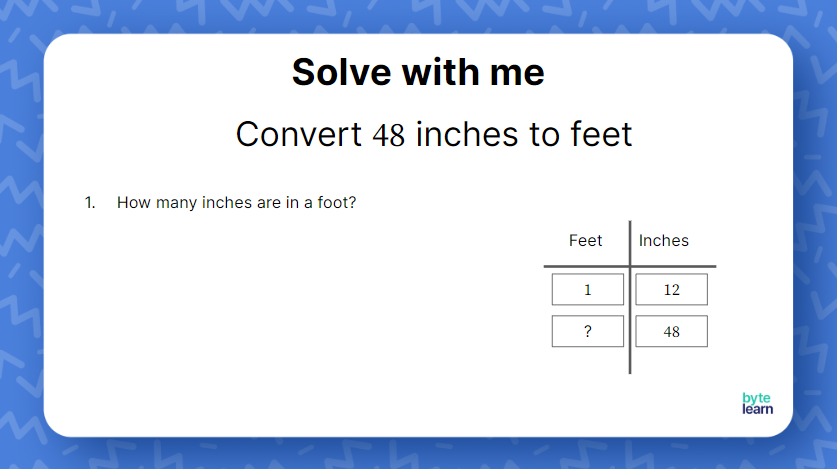ResourcesTestimonials
Plans
ResourcesTestimonials
PlansLesson plan

# Converting Measurements Lesson Plan

## Overview

In this lesson, students will convert measurements by creating ratio tables. They will work on one unit and two unit conversions. They start the lesson with a warmup on reviewing a few different conversions and to notice that they will need to use the unit rate in order to find the conversion. This lesson should take one-45 minute class period.

Ratios And Rates
6.RP.A.3.D

## Objective

Students will be able to convert units using ratio reasoning.

## Materials

• Teacher Slideshow
• Warm-up Each student will need a half sheet of paper
• Partner Activity Card Sort Each group of two will need a card sort
• Scissors
• Online Practice

## How to Teach Converting Measurements

### Warm-up

Start the class period by giving each student the warmup. This is a good short activity for students to see which unit conversions they already know. Give students a few minutes to match up all the units.Once students are done, go over all of the matches. Likely, between all the students, you will be able to put each match together. Ask students what they noticed about all of the matches. Students should notice that each match has a “1” in their ratio. Explain to students that when converting measurements, they will need to start out by finding the ratio to convert the unit.

### First example

Transition into the lesson by telling students they will create ratio tables for converting units and converting unit word problems. Slides 1-8 will allow you to take students through the process of using a ratio table to convert 48 inches to feet.### Create a ratio table

So to start, ask students how many inches are in a foot. We’ll use their answer to create the ratio table shown on slide 3 below:### Find the multiplier/divisor

Next, you’ll want to ask what multiplier or divisor would get you from the left side to the right side. You can rephrase this question and ask, “what would we need to multiply 1 by to get to 12?” Students should recognize that we would need to multiply by 12.

### Create an equivalent ratio

Then lastly we want to create an equivalent ratio, meaning we’ll always want the multiplier from left to right to be 12. So ask students, “from left to right we need to multiply by 12, so what would we need to do to go from right to left?” The phrasing of this question may have students thinking about inverse operations or the inverse of 12. You can have students consult with a partner before asking for anyone wanting to try to explain to the class. Slide 7 shows that if we multiply by 12 to go from left to right, we should divide by 12 to go from right to left.

Now it’s as easy as dividing 48 by 12 to get 3 feet!

### More examples as a class

The teacher slideshow contains a few more example problems to do with the whole class. Follow the same process as the first example as you set up and complete the ratio table to find the conversions.

### Partner activity

Hand out to students the partner activity where they will do a card sort to match the different conversions. Remind students to use a ratio table if they get stuck. Have students show all work on a separate sheet of notebook paper for when you go around to check the matches. If students have work shown, they can explain why or how they got to their answer.

## Converting Measurements Practice

After students have completed their lesson, it’s time for some independent practice! ByteLearn gives you access to tons of converting measurements activities. Check out the online practice and assign to your students for classwork and/or homework!Converting Measurements Practice